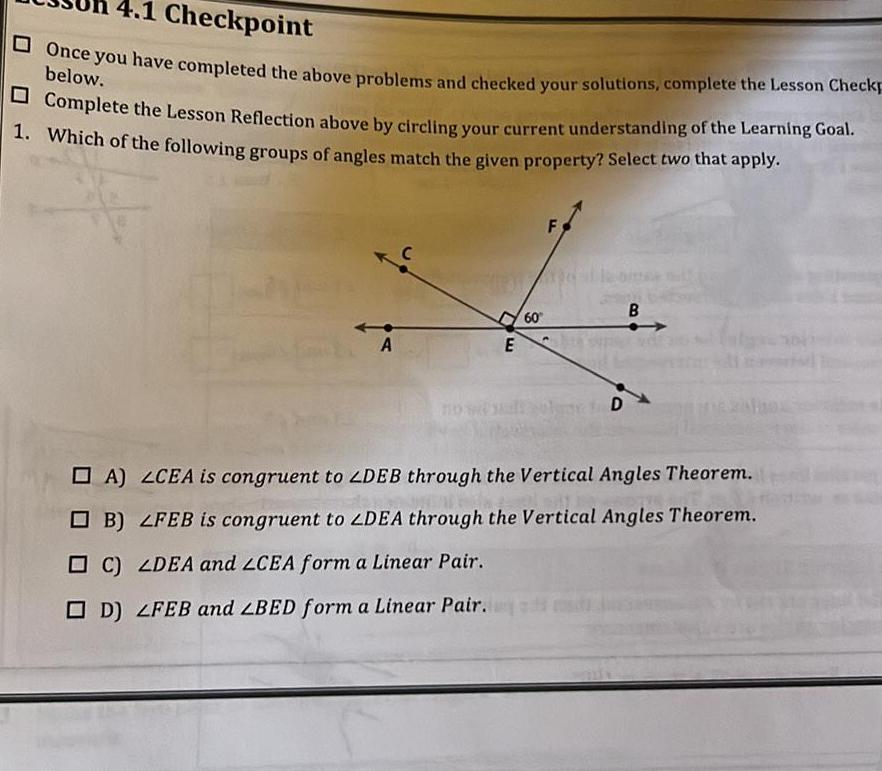Question:

# 4 1 Checkpoint Once you have completed the above problems

Last updated: 11/20/20234 1 Checkpoint Once you have completed the above problems and checked your solutions complete the Lesson Checkp below Complete the Lesson Reflection above by circling your current understanding of the Learning Goal 1 Which of the following groups of angles match the given property Select two that apply A E C LDEA and LCEA form a Linear Pair D LFEB and BED form a Linear Pair 60 F D B A LCEA is congruent to ZDEB through the Vertical Angles Theorem B LFEB is congruent to ZDEA through the Vertical Angles Theorem